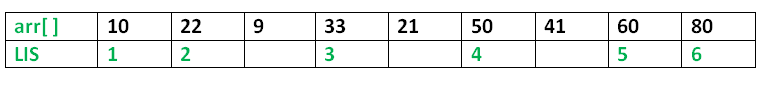Java Program for Longest Increasing Subsequence

The Longest Increasing Subsequence (LIS) problem is to find the length of the longest subsequence of a given sequence such that all elements of the subsequence are sorted in increasing order. For example, the length of LIS for {10, 22, 9, 33, 21, 50, 41, 60, 80} is 6 and LIS is {10, 22, 33, 50, 60, 80}.More Examples:

Input  : arr[] = {3, 10, 2, 1, 20}
Output : Length of LIS = 3
The longest increasing subsequence is 3, 10, 20

Input  : arr[] = {3, 2}
Output : Length of LIS = 1
The longest increasing subsequences are {3} and {2}

Input : arr[] = {50, 3, 10, 7, 40, 80}
Output : Length of LIS = 4
The longest increasing subsequence is {3, 7, 40, 80}

Recommended: Please try your approach on {IDE} first, before moving on to the solution.

Optimal Substructure:
Let arr[0..n-1] be the input array and L(i) be the length of the LIS ending at index i such that arr[i] is the last element of the LIS.
Then, L(i) can be recursively written as:
L(i) = 1 + max( L(j) ) where 0 < j < i and arr[j] < arr[i]; or
L(i) = 1, if no such j exists.
To find the LIS for a given array, we need to return max(L(i)) where 0 < i < n.
Thus, we see the LIS problem satisfies the optimal substructure property as the main problem can be solved using solutions to subproblems.

Following is a simple recursive implementation of the LIS problem. It follows the recursive structure discussed above.

 /* A Naive Java Program for LIS Implementation */ class LIS {     static int max_ref; // stores the LIS        /* To make use of recursive calls, this function must return    two things:    1) Length of LIS ending with element arr[n-1]. We use       max_ending_here for this purpose    2) Overall maximum as the LIS may end with an element       before arr[n-1] max_ref is used this purpose.    The value of LIS of full array of size n is stored in    *max_ref which is our final result */     static int _lis(int arr[], int n)     {         // base case         if (n == 1)             return 1;            // 'max_ending_here' is length of LIS ending with arr[n-1]         int res, max_ending_here = 1;            /* Recursively get all LIS ending with arr, arr ...            arr[n-2]. If   arr[i-1] is smaller than arr[n-1], and            max ending with arr[n-1] needs to be updated, then            update it */         for (int i = 1; i < n; i++) {             res = _lis(arr, i);             if (arr[i - 1] < arr[n - 1] && res + 1 > max_ending_here)                 max_ending_here = res + 1;         }            // Compare max_ending_here with the overall max. And         // update the overall max if needed         if (max_ref < max_ending_here)             max_ref = max_ending_here;            // Return length of LIS ending with arr[n-1]         return max_ending_here;     }        // The wrapper function for _lis()     static int lis(int arr[], int n)     {         // The max variable holds the result         max_ref = 1;            // The function _lis() stores its result in max         _lis(arr, n);            // returns max         return max_ref;     }        // driver program to test above functions     public static void main(String args[])     {         int arr[] = { 10, 22, 9, 33, 21, 50, 41, 60 };         int n = arr.length;         System.out.println("Length of lis is "                            + lis(arr, n) + "\n");     } } /*This code is contributed by Rajat Mishra*/

Output:

Length of lis is 5

Overlapping Subproblems:
Considering the above implementation, following is recursion tree for an array of size 4. lis(n) gives us the length of LIS for arr[].

lis(4)
/        |
lis(3)    lis(2)   lis(1)
/           /
lis(2) lis(1) lis(1)
/
lis(1)

We can see that there are many subproblems which are solved again and again. So this problem has Overlapping Substructure property and recomputation of same subproblems can be avoided by either using Memoization or Tabulation. Following is a tabluated implementation for the LIS problem.

 /* Dynamic Programming Java implementation of LIS problem */    class LIS {     /* lis() returns the length of the longest increasing        subsequence in arr[] of size n */     static int lis(int arr[], int n)     {         int lis[] = new int[n];         int i, j, max = 0;            /* Initialize LIS values for all indexes */         for (i = 0; i < n; i++)             lis[i] = 1;            /* Compute optimized LIS values in bottom up manner */         for (i = 1; i < n; i++)             for (j = 0; j < i; j++)                 if (arr[i] > arr[j] && lis[i] < lis[j] + 1)                     lis[i] = lis[j] + 1;            /* Pick maximum of all LIS values */         for (i = 0; i < n; i++)             if (max < lis[i])                 max = lis[i];            return max;     }        public static void main(String args[])     {         int arr[] = { 10, 22, 9, 33, 21, 50, 41, 60 };         int n = arr.length;         System.out.println("Length of lis is " + lis(arr, n) + "\n");     } } /*This code is contributed by Rajat Mishra*/

Output:

Length of lis is 5

Please refer complete article on Dynamic Programming | Set 3 (Longest Increasing Subsequence) for more details!

My Personal Notes arrow_drop_up